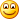# Linq distinct with lambda

Linq Distinct function accepts an IEqualityComparer<T> used to compare values of the IEnumerable to remove equal objects to implement the Distinct() function. Sometimes it is more useful to specify a simple Func<T, T, Boolean>, a simple predicate that will implement the concept of Equality between elements. To support such a scenario you can write this simple Extension method.

 ``````1 2 3 4 5 6 `````` ``````public static IEnumerable Distinct( this IEnumerable source, Func comparer) { return new DistinctByLambda(comparer, source); } ``````

All the work is done by the DistinctByLambda class, that accepts a reference to the original IEnumerable sequence of elements and the predicate to verify for equality. Here is the code.

 `````` 1 2 3 4 5 6 7 8 9 10 11 12 13 14 15 16 17 18 19 20 21 22 23 24 25 26 27 28 29 30 `````` ``````public class DistinctByLambda : IEnumerable { private Func _comparer;   private IEnumerable _source;   public DistinctByLambda(Func comparer, IEnumerable source) { _comparer = comparer; _source = source; }   public IEnumerator GetEnumerator() { List alreadyFound = new List(); foreach (T element in _source) { if (!alreadyFound.Exists(el => _comparer(el, element))) { alreadyFound.Add(element); yield return element; } } }   IEnumerator IEnumerable.GetEnumerator() { return GetEnumerator(); } } ``````

The code is really simple, the IEnumerable.GetEnumerator function is implemented with a simple internal list that stores all distinct elements. For each element of the original list, if it is not equal to any element of the original list it is stored in the list and then returned to the caller; if the element is equal to one element in the list, it gets not returned.

You can use the following function in this simple way.

 ``````1 2 3 4 5 6 7 8 `````` ``````[Test] public void VerifyDistinctByLambda() { IList list = new List() {new Test("a", 11), new Test("a", 12), new Test("b", 12)}; var result = list.Distinct((t1, t2) => t1.BlaBla == t2.BlaBla); Assert.That(result.Count(), Is.EqualTo(2)); Assert.That(result, Is.EquivalentTo(new List() {new Test("a", 11), new Test("b", 12)})); } ``````

I use a simple Test class that contains two properties, and call Distinct with a  function that compare only the BlaBla property. Now you can use lambda with distinctAlk.